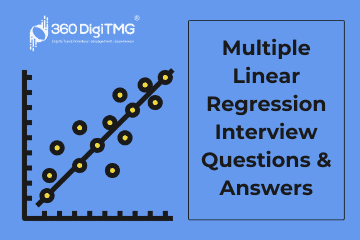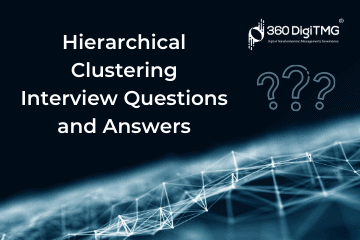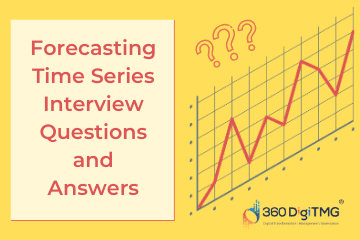Home / Blog / Interview Questions on Data Science / Multiple Linear Regression Interview Questions & Answers

# Multiple Linear Regression Interview Questions & Answers

• September 07, 2022
• 8465
• 37### Meet the Author : Mr. Bharani Kumar

Bharani Kumar Depuru is a well known IT personality from Hyderabad. He is the Founder and Director of Innodatatics Pvt Ltd and 360DigiTMG. Bharani Kumar is an IIT and ISB alumni with more than 17 years of experience, he held prominent positions in the IT elites like HSBC, ITC Infotech, Infosys, and Deloitte. He is a prevalent IT consultant specializing in Industrial Revolution 4.0 implementation, Data Analytics practice setup, Artificial Intelligence, Big Data Analytics, Industrial IoT, Business Intelligence and Business Management. Bharani Kumar is also the chief trainer at 360DigiTMG with more than Ten years of experience and has been making the IT transition journey easy for his students. 360DigiTMG is at the forefront of delivering quality education, thereby bridging the gap between academia and industry.

• ### This model considers the fewest number of predictor/explanatory variables, in comparison with other models, which are nearly equivalent in terms of significance and fit

• a) Law of MLR
• b) Law of equations
• c) Law of Parsimony
• d) Law of Parsinomy

Answer - c) Law of Parsimony

• ### In MLR, if there are three variables, what would be the shape?

• a) Circle
• b) Hyperplane
• c) Triangle
• d) None of the above

• a) True
• b) False

• a) True
• b) False

• ### To visualize all the pair of variables at a time, we use

• a) Scatter plot
• b) Box plot
• c) Scatter plot matrix
• d) None of the above

Answer - c) Scatter plot matrix

• ### Reducing the number of features and computational complexity of the model is done by

• a) Feature selection
• b) Cross selection
• c) Complete selection
• d) None of the above

• ### In regression ________ are the feature selection methods used .

• a) Forward selection
• b) Backward elimination
• c) Stepwise regression
• d) All the above

Answer - d) All the above

• ### We use the ______ plot to know whether there is a relationship between two variables.

• a) Histogram
• b) Box plot
• c) Scatter plot
• d) Bar plot

• ### After fitting the model with all the required variables______ method is used for identifying and removing independent variables that do not contribute enough to the model.

• a) Forward selection
• b) Backward elimination
• c) Stepwise regression
• d) All the above

• ### Considering variables one by one and building the model by checking the significance value & R square is done by using _____ method.

• a) Stepwise Regression
• b) Backward elimination
• c) Both b and c
• d) Stepwise elimination

• a) True
• b) False

• ### ____________increases only when independent variable is significant and affects dependent variable.

• a) R-squared
• c) Both the above
• d) None of the above

• ### R square=

• a) SSR/SSE
• b) SSE/SSR
• c) SSS/SEE
• d) SSR/SST

Answer - d) (Sum Squared Regression (SSR)/Total Sum of Squares (SST)

• ### A lower AIC or BIC value indicates a _______ fit.

• a) Worst fit
• b) Better fit
• c) Low fit
• d) None of the above

• a) Yes
• b) No

### Data Science Training Institutes in Other Locations